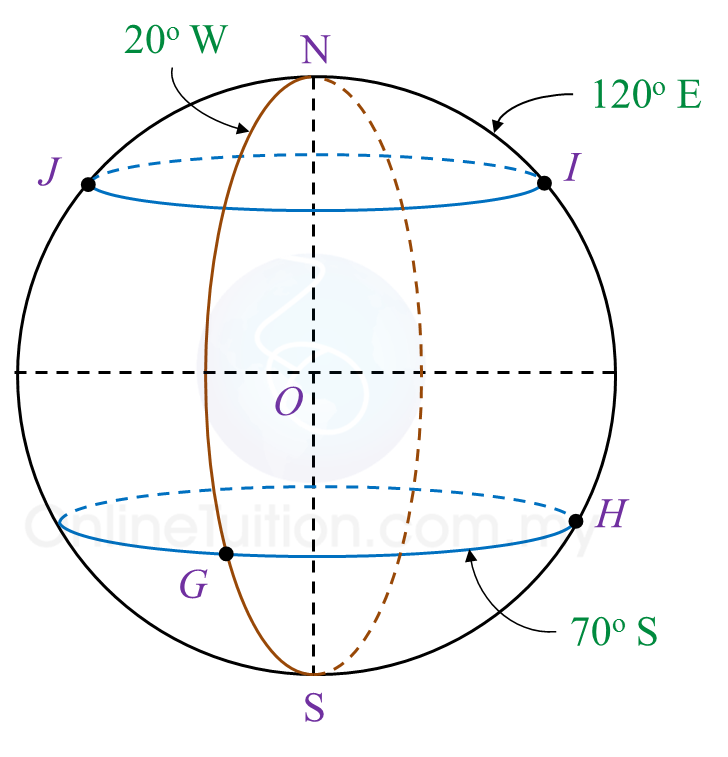# 9.6 SPM Practice (Long Questions)

Question 8 (12 marks):
Diagram 8 shows four points, G, H, I and J on the surface of the Earth. JI is the diameter of the parallel of latitude 50o N. O is the centre of the Earth.Diagram 8

(a)
State the location of G.

(b) Calculate the shortest distance, in nautical mile, from I to J measured along the surface of the Earth.

(c) Calculate the shortest distance, in nautical mile, from G to H measured along the common parallel of latitude.

(d) An aeroplane took off from I and flew due south to point P. The average speed of the journey was 800 knots. The time taken for the flight was 5.25 hours.
Calculate the latitude of P.

Solution:
(a)
Location of G = (70o S, 20o W)

(b)
∠ JOI
= 180o – 50o – 50o
= 80o
Distance of I to J
= 80o × 60’
= 4800 nautical miles

(c)
Distance of G to H
= (20o + 120o) × 60’ × cos 70o
= 140o × 60’ × cos 70o
= 2872.97 nautical miles

(d)